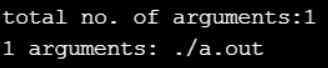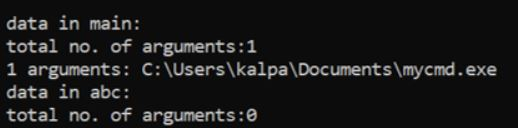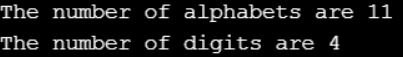# Command Line Arguments in C

## Command Line Arguments in C Language with Examples

In this article, I am going to discuss the Command Line Arguments in C Language with Examples. Please read our previous articles, where we discussed Pointers in C. As part of this article, you will learn what is Command Line Arguments in C Language, its type, and when and how to use Command Line Arguments in C Program with examples.

##### Command Line Arguments in C Language:

It is a procedure of passing the arguments to the main function from the command prompt. By using command-line arguments, we can create user-defined commands. In the implementation, when we were required to develop an application for the DOS operating system then recommended going for command-line arguments. DOS is a character-based or command-based OS i.e. if we are required to perform any task, we need to use the specific commands only. When we are developing the program in command-line arguments, then the main function will take 2 arguments i.e. argc & argv i.e. main (int argc, char *argv[])

The argc is a variable of type an integer, it maintains the total number of arguments count value. The argv is a variable of type char*, which maintains actual argument values that are passed to the main() function. In C and C++, by default total number of arguments is 1, i.e. programname.exe

##### Program to understand Command Line Arguments in C Language:
```#include <stdio.h>
int main (int argc, char *argv[])
{
int i;
printf ("\n total no. of arguments:%d", argc);
for (i = 0; i < argc; i++)
printf ("\n %d arguments: %s", i + 1, argv[i]);
return 0;
}
```
###### Output:##### Command Line Arguments Example in C Language:
```#include <stdio.h>
int main (int argc, char *argv[])
{
int i;
printf ("\n total no. of arguments:%d", argc);
for (i = 0; i < argc; i++)
printf ("\n %d arguments: %s", i + 1, argv[i]);
return 0;
}
```

//save the file as mytest.c
//compile mytest.c
//Build or rebuild mytest.c

Load the command prompt & change the directory to a specific location where the application file is available. To execute the program, just we required to use the program name or programname.exe. Command-line arguments related programs are not recommended to execute by IDE because we can’t pass the arguments to the main function.

argc & argv are local variable to main() so command prompt related data outside of main(), then recommended to go for aargc & aargv variables, which is defined in dos.h

##### Program to understand Command Line Arguments in C Language:
```#include <stdio.h>
#include<dos.h>
void abc ()
{
int i, aargc;
char *aargv[i];
printf ("\n data in abc:");
printf ("\n total no. of arguments:%d", aargc);
for (i = 0; i < aargc; i++)
printf ("\n %d arguments: %s", i + 1, aargv[i]);
}

int main (int argc, const char *argv[])
{
int i;
printf ("\n data in main:");
printf ("\n total no. of arguments:%d", argc);
for (i = 0; i < argc; i++)
printf ("\n %d arguments: %s", i + 1, argv[i]);
abc ();
return 0;
}
```
###### Output:By using command-line arguments, it is possible to pass any type of data but all are treated like strings only. When we are working with command line arguments, always recommended to convert string type to numeric type properly before the execution of the logic. Conversion related all predefined functions are declared in stdlib.

##### Stdlib related predefined functions are:

atoi(), atof(), atol(), _atold(), itoa(), ltoa(), abort(), exit(), _exit(), _c_exit(), atexit(), raise(), signal(), min(), max(), random(), randomize(), rand(), srand(), abs(), labs(), strtod(), strtol(), strtold(), strtoul(), wctomb(), wcstombs(), fcvt(), gcvt(), lfind(), lsearch(), _lrotl(), _lrotr(), div(), ldiv()

Constants: EXIT_SUCCESS, EXIT_FAILURE

atoi(): By using this, we can convert a string into an integer type. atoi() functions requires 1 arguments of type char* & returns int type.

Syntax: int atoi (const char*str);

atol(): By using this, we can convert a string into a long integer type.

Syntax: long atol (const char*str);

atof(): By using this predefined function, we can convert a string into a double data type.

Syntax: double atof (const char*str);

_atold(): By using this predefined function, we can convert a string into a long double type.

Syntax: long double _atold (const char*str);

By using fcvt(), gcvt(), ecvt(), we can convert float to string type.

##### Program:
```#include <stdio.h>
int myatoi (char *str)
{
int res = 0;
for (int i = 0; str[i] != '\0'; ++i)
res = res * 10 + str[i] - '0';
return res;
}

int main ()
{
char str[] = "89789";
int val = myatoi (str);
printf ("%d ", val);
return 0;
}
```

Output: 89789

##### <ctype.h>:

Character datatype specific functions are available in ctype.h. ctype.h related predefined functions are:

1. isalpha(): checking alphabet or not.
2. isascii(): checking input data within the ASCII range or not.
3. iscntrl(): if control button from keyboard.
4. isspace(): if space button from keyboard.
5. isupper(): required to use from A to Z.
6. isdigit(): checking whether the numeric type or not.
7. islower(): to check if it is a-z.
8. tolower(): convert uppercase to lowercase.
9. toupper(): convert lowercase to uppercase.
10. toascii(): convert to ASCII value.

When the function prefix ‘is’ available, then it returns Boolean type i.e. true or false. If ‘to’ is available, then it returns an integer value.

##### Program:
```#include <stdio.h>
#include <ctype.h>
void identify_alpha_numeric (char a[])
{
int count_alpha = 0, count_digit = 0;
for (int i = 0; a[i] != '\0'; i++)
{
if (isalpha (a[i]))
count_alpha++;
if (isdigit (a[i]))
count_digit++;
}
printf ("The number of alphabets are %d\n", count_alpha);
printf ("The number of digits are %d", count_digit);
}

int main ()
{
char a[] = "Hi 1996, " " How Are You?";
identify_alpha_numeric (a);
}
```
###### Output: